Dave's Math Tables: Algebraic Graphs(Math | OddsEnds | Graphs | Algebra)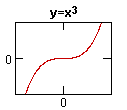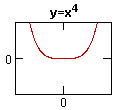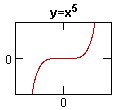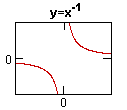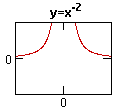Conic Sections(see also Conic Sections) Point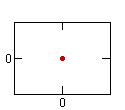x^2 + y^2 = 0 Circle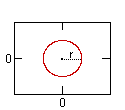x^2 + y^2 = r^2 Ellipse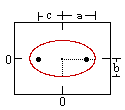x^2 / a^2 + y^2 / b^2 = 1 Ellipse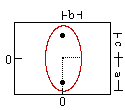x^2 / b^2 + y^2 / a^2 = 1 Hyperbola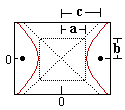x^2 / a^2 - y^2 / b^2 = 1 Parabola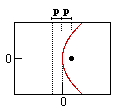4px = y^2 Parabola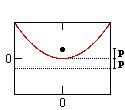4py = x^2 Hyperbola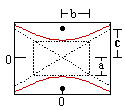y^2 / a^2 - x^2 / b^2 = 1 For any of the above with a center at (j, k) instead of (0,0), replace each x term with (x-j) and each y term with (y-k) to get the desired equation.